Sign in# 9 Simple Math Tricks You’ll Wish You Had Always Known

It is not totally your fault if you cannot solve these math problems in a simpler and faster way. Maybe it is because you don't know how to simplify any math question. Many of you will be now familiar with math tricks as a result of this post, as we have provided you with a list of them.

1. If you want to remember Pi, there are a few things you can do.2. When you are multiplying by 6, 7, 8, and 9 using your hands only, follow the step below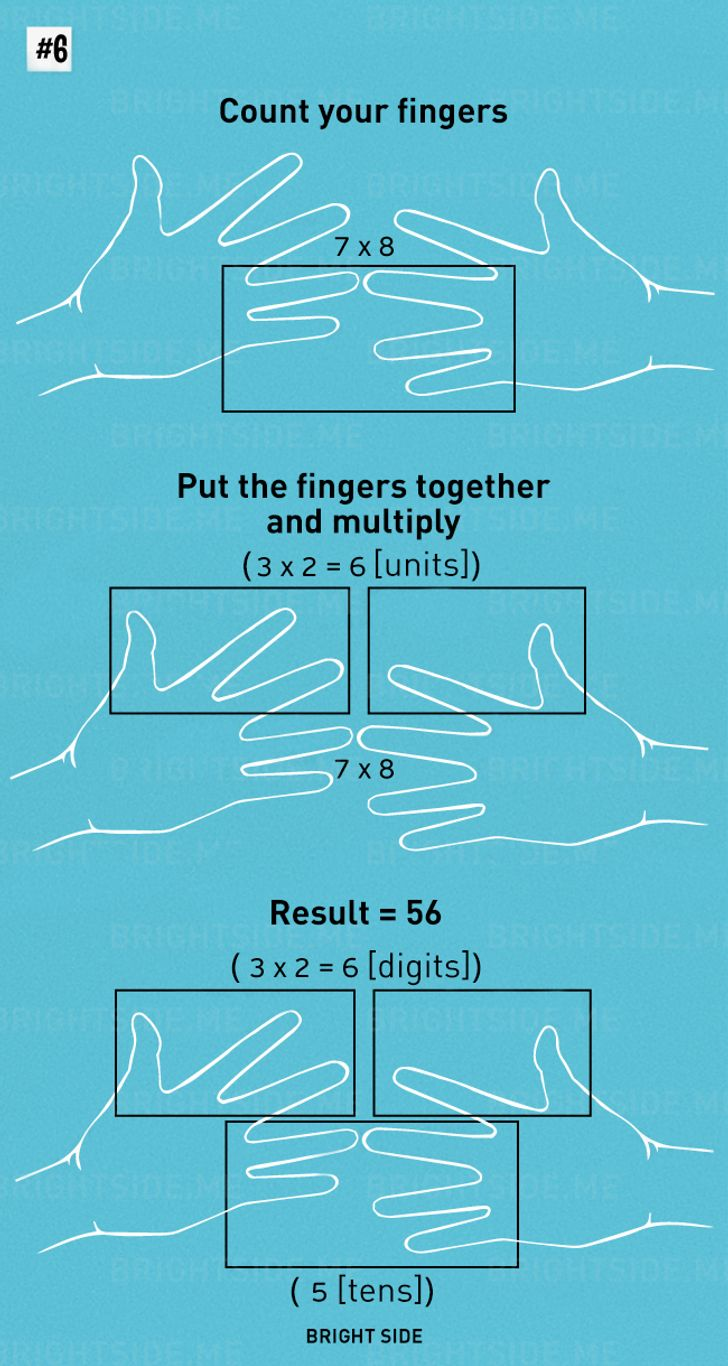3. Follow this method to calculate the percentage of a number.4. Quickly multiplying significant numbers in your head.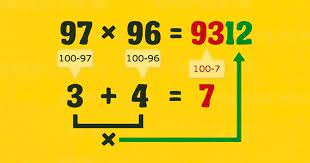5. Would you like to learn the 'nine times' table? Follow this procedure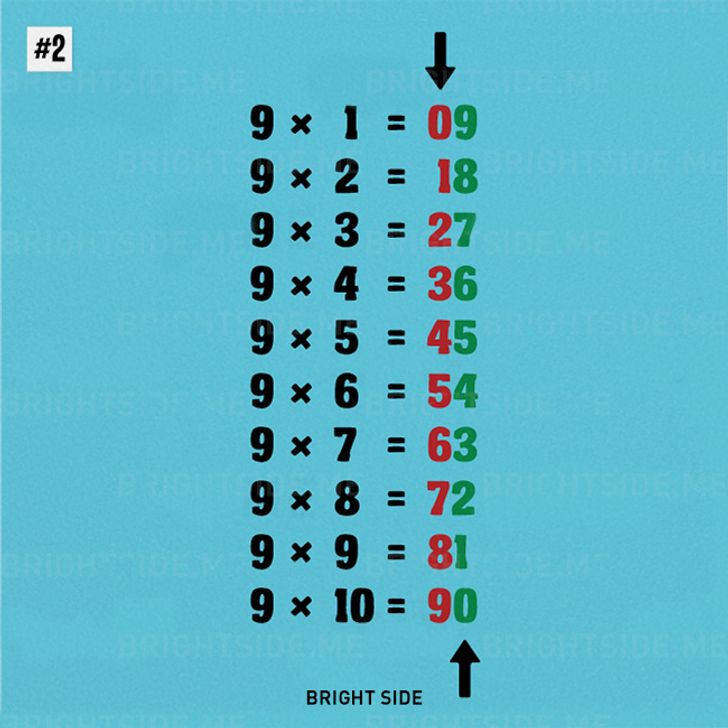6. The easiest way to add and subtract fractions is through the butterfly method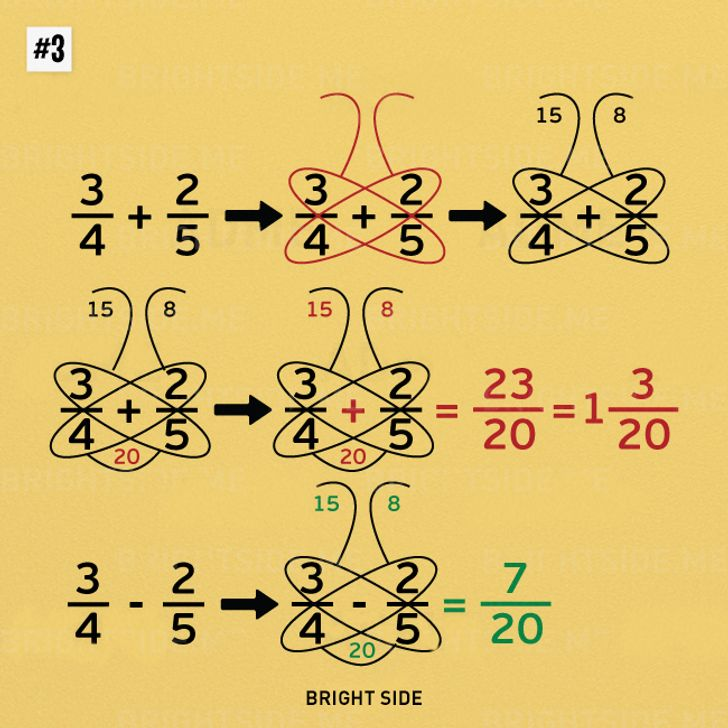7. The technique below is effective for finding a fraction of a whole number.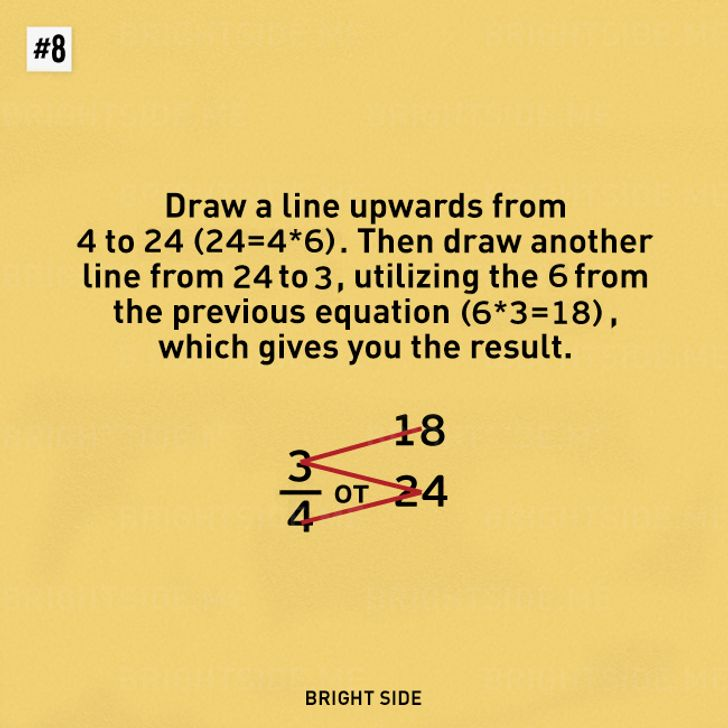8. When changing values from Celsius to Fahrenheit and vice versa, follow these steps.9. Use this example to multiply a number by 11: E.g. 11 x 32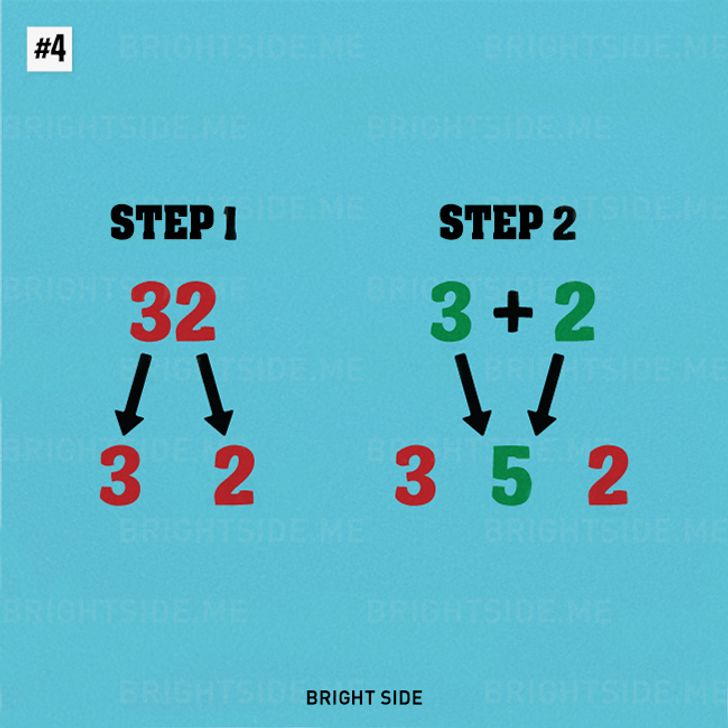# Photos: Courtesy of shutterstock

Content created and supplied by: nakurunews (via Opera News )

Pi

COMMENTS

Load app to read more comments